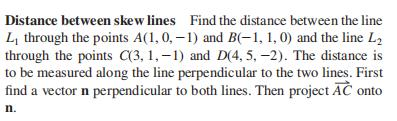### Still have math questions?Distance between skew lines Find the distance between the line $$L _ { 1 }$$ through the points $$A ( 1,0 , - 1 )$$ and $$B ( - 1,1,0 )$$ and the line $$L _ { 2 }$$ through the points $$C ( 3,1 , - 1 )$$ and $$D ( 4,5 , - 2 )$$ . The distance is to be measured along the line perpendicular to the two lines. First find a vector $$n$$ perpendicular to both lines. Then project $$\vec { A C }$$ onto $$n$$ .
$$11 / \sqrt { 107 }$$